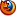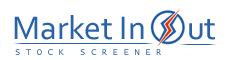Please enable JavaScript to view this page content properly Log In | Sign Up
 RSI Stochastic Momentum CCI Chaikin Oscillator Aroon Oscillator Williams' %R MFI CMF CRSI TMF TRIX TSI Ultimate Oscillator Rate Of Change Balance Of Power Awesome Oscillator Accelerator Oscillator Stochastic RSI STC RMI RCI SMA of RSI EMA of RSI SMI SMIE CHMO
 Oscillators are useful for identifying short-term movements. They determine turning points useful in identifying buy/sell opportunities.
 RSI Action RSI(14) Bullish Divergence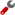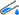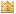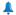RSI(14) Crossed Above 70RSI(14) Crossed Above 50RSI(14) Crossed Above 30RSI(14) Trending Up Last 3 DaysRSI(14) Trending Up Last 7 DaysRSI(14) Trending Up Last 13 DaysRSI(14) Trending Up Last 26 DaysRSI(14) Between 70 and 100RSI(14) Between 50 and 70RSI(14) Between 30 and 50RSI(14) Between 0 and 30RSI(14) Trending Down Last 26 DaysRSI(14) Trending Down Last 13 DaysRSI(14) Trending Down Last 7 DaysRSI(14) Trending Down Last 3 DaysRSI(14) Crossed Below 30RSI(14) Crossed Below 50RSI(14) Crossed Below 70RSI(14) Bearish Divergence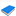Relative Strength Index (RSI) - Technical Analysis from A to Z The Relative Strength Index ("RSI") is a popular oscillator. It was first introduced by Welles Wilder in an article in Commodities Magazine in June, 1978. When Wilder introduced the RSI, he recommended using a 14-day RSI. Since then, the 9-day and 25-day RSIs have also gained popularity. Because you can vary the number of time periods in the RSI calculation, I suggest that you experiment to find the period that works best for you. The fewer days used to calculate the RSI, the more volatile the indicator.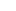Best viewed in